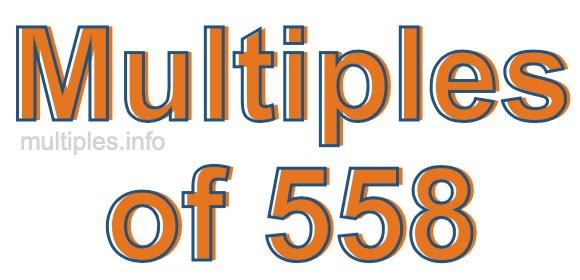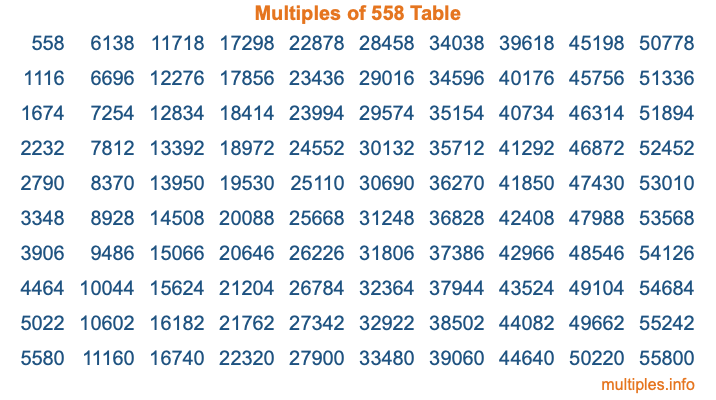Multiples of 558Welcome to the Multiples of 558 page. Here we will first teach you everything you will ever need to know about the multiples of 558, and then give you a study guide summary of everything we taught you to make sure you remember it all. Use this page to look up facts and learn information about the multiples of 558. This page will make you a multiples of five hundred fifty-eight expert!

Definition of Multiples of 558
Multiples of 558 are all the numbers that when divided by 558 equal an integer. Each of the multiples of 558 are called a multiple. A multiple of 558 is created by multiplying 558 by an integer.

Therefore, to create a list of multiples of 558, you start with 1 multiplied by 558, then 2 multiplied by 558, then 3 multiplied by 558, and so on for as long as you want. Thus, the list of the first five multiples of 558 is 558, 1116, 1674, 2232, and 2790. To see a larger list of multiples of 558, see the printable image of Multiples of 558 further down on this page. We also have a category where you can choose any nth multiple of 558.

Multiples of 558 Checker
The Multiples of 558 Checker below checks to see if any number of your choice is a multiple of 558. In other words, it checks to see if there is any number (integer) that when multiplied by 558 will equal your number. To do that, we divide your number by 558. If the the quotient is an integer, then your number is a multiple of 558.

Is  a multiple of 558?

Least Common Multiple of 558 and ...
A Least Common Multiple (LCM) is the lowest multiple that two or more numbers have in common. This is also called the smallest common multiple or lowest common multiple and is useful to know when you are adding our subtracting fractions. Enter one or more numbers below (558 is already entered) to find the LCM.

Check out our LCM Calculator if you need more details about the Least Common Multiple or if you need the LCM for different numbers for adding and subtraction fractions.

nth Multiple of 558
As we stated above, 558 is the first multiple of 558, 1116 is the second multiple of 558, 1674 is the third multiple of 558, and so on. Enter a number below to find the nth multiple of 558.

th multiple of 558

Multiples of 558 vs Factors of 558
558 is a multiple of 558 and a factor of 558, but that is where the similarities end. All postive multiples of 558 are 558 or greater than 558. All positive factors of 558 are 558 or less than 558.

Below is the beginning list of multiples of 558 and the factors of 558 so you can compare:

Multiples of 558: 558, 1116, 1674, 2232, 2790, etc.

Factors of 558: 1, 2, 3, 6, 9, 18, 31, 62, 93, 186, 279, 558

As you can see, the multiples of 558 are all the numbers that you can divide by 558 to get a whole number. The factors of 558, on the other hand, are all the whole numbers that you can multiply by another whole number to get 558.

It's also interesting to note that if a number (x) is a factor of 558, then 558 will also be a multiple of that number (x).

Multiples of 558 vs Divisors of 558
The divisors of 558 are all the integers that 558 can be divided by evenly. Below is a list of the divisors of 558.

Divisors of 558: 1, 2, 3, 6, 9, 18, 31, 62, 93, 186, 279, 558

The interesting thing to note here is that if you take any multiple of 558 and divide it by a divisor of 558, you will see that the quotient is an integer.

Multiples of 558 Table
Below is an image of the first 100 multiples of 558 in a table. The table is in chronological order, column by column. The first column has the first ten multiples of 558, the second column has the next ten multiples of 558, and so on.The Multiples of 558 Table is also referred to as the 558 Times Table or Times Table of 558. You are welcome to print out our table for your studies.

Negative Multiples of 558
Although not often discussed or needed in math, it is worth mentioning that you can make a list of negative multiples of 558 by multiplying 558 by -1, then by -2, then by -3, and so on, to get the following list of negative multiples of 558:

-558, -1116, -1674, -2232, -2790, etc.

Multiples of 558 Summary
Below is a summary of important Multiples of 558 facts that we have discussed on this page. To retain the knowledge on this page, we recommend that you read through the summary and explain to yourself or a study partner why they hold true.

There are an infinite number of multiples of 558.

A multiple of 558 divided by 558 will equal a whole number.

558 divided by a factor of 558 equals a divisor of 558.

The nth multiple of 558 is n times 558.

The largest factor of 558 is equal to the first positive multiple of 558.

558 is a multiple of every factor of 558.

558 is a multiple of 558.

A multiple of 558 divided by a divisor of 558 equals an integer.

558 divided by a divisor of 558 equals a factor of 558.

Any integer times 558 will equal a multiple of 558.

Multiples of a Number
Here you can get the multiples of another number, all with the same attention to detail as we did for multiples of 558 on this page.

Multiples of
Multiples of 559
Did you find our page about multiples of five hundred fifty-eight educational? Do you want more knowledge? Check out the multiples of the next number on our list!